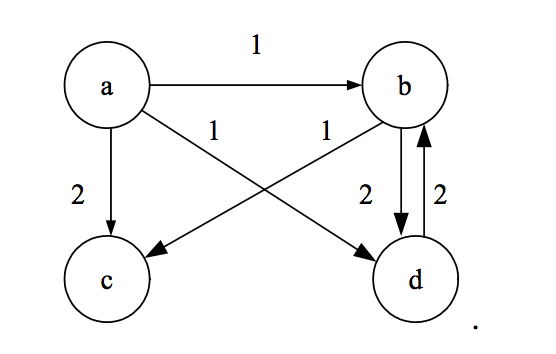### Introduction

Unlike the queue, the stack and the linked list, the graph is a non-linear data structure, similar to the tree. It is composed of a collection of nodes which are connected to each other. The connections are called edges and can be either directed or non-directed. The edges can also have weights associated to them. These could represent distances, times or any other kind of information which describes that connection.### Implementation

As with the other data structures, there are several types of graphs and I had to make a choice here. Eventually I decided to implement a weighted directed graph. This means that every edge is going to have a direction and a weight associated to it.

To start with we created a struct which will hold the references to all the nodes in the graph. The order of nodes in this slice is going to be important because the index of the graph is going to also be its ID.

``````// Graph : represents a Graph
type Graph struct {
nodes []*GraphNode
}
``````

Next we created a struct for an individual node. A node will have an `id` field and an `edges` field which is a map of node IDs and weights.

So, if node `0` is linked to node `1`, with an edge of weight `7` then we are going to add to the `edges` map a key `1` with the value `7`.

``````// GraphNode : represents a Graph node
type GraphNode struct {
id    int
edges map[int]int
}
``````

As always we add a constructor function.

``````// New : returns a new instance of a Graph
func New() *Graph {
return &Graph{
nodes: []*GraphNode{},
}
}
``````

Now we are going to add the following methods: `AddNode`, `AddEdge`, `Neighbors`, `Nodes` and `Edges`.

The `AddNode` method is going to add a new node at the first empty position of the Graph’s nodes field, which is going to be equal to the length of this field. The return value is going to be the new `id`.

``````// AddNode : adds a new node to the Graph
func (g *Graph) AddNode() (id int) {
id = len(g.nodes)
g.nodes = append(g.nodes, &GraphNode{
id:    id,
edges: make(map[int]int),
})
return
}
``````

For `AddEdge` we have to pass the IDs of two nodes in the order that we want the direction of the edge to be in, and the edge’s weight.

``````// AddEdge : adds a directional edge together with a weight
func (g *Graph) AddEdge(n1, n2 int, w int) {
g.nodes[n1].edges[n2] = w
}
``````

Next, the `Neighbors` method is going to iterate through all nodes and their edges to find the neighbors of a specific node ID. This is the most expensive function of all.

``````// Neighbors : returns a list of node IDs that are linked to this node
func (g *Graph) Neighbors(id int) []int {
neighbors := []int{}
for _, node := range g.nodes {
for edge := range node.edges {
if node.id == id {
neighbors = append(neighbors, edge)
}
if edge == id {
neighbors = append(neighbors, node.id)
}
}
}
return neighbors
}
``````

Then we create a method `Nodes` that is going to list the IDs of all nodes.

``````// Nodes : returns a list of node IDs
func (g *Graph) Nodes() []int {
nodes := make([]int, len(g.nodes))
for i := range g.nodes {
nodes[i] = i
}
return nodes
}
``````

And finally, we create the `Edges` method which is going to list all the edges as a slice of arrays, which contain the ID of the start node, the ID of the end node and the weight of the edge.

``````// Edges : returns a list of edges with weights
func (g *Graph) Edges() []int {
edges := make([]int, 0, len(g.nodes))
for i := 0; i < len(g.nodes); i++ {
for k, v := range g.nodes[i].edges {
edges = append(edges, int{i, k, int(v)})
}
}
return edges
}
``````

### Conclusion

A good next step when studying graphs is to also implement a search/traversal method. You can choose between BFS (breadth first search) and DFS (depth first search). But I will leave this to you ;)

### Source code with tests

https://github.com/dorin131/go-data-structures